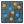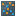You are viewing the RapidMiner Studio documentation for version 8.0 - Check here for latest version

#K-Means (Kernel) (RapidMiner Studio Core)

## Synopsis

This operator performs clustering using the kernel k-means algorithm. Clustering is concerned with grouping objects together that are similar to each other and dissimilar to the objects belonging to other clusters. Kernel k-means uses kernels to estimate the distance between objects and clusters. K-means is an exclusive clustering algorithm.

## Description

This operator performs clustering using the kernel k-means algorithm. The k-means is an exclusive clustering algorithm i.e. each object is assigned to precisely one of a set of clusters. Objects in one cluster are similar to each other. The similarity between objects is based on a measure of the distance between them. Kernel k-means uses kernels to estimate the distance between objects and clusters. Because of the nature of kernels it is necessary to sum over all elements of a cluster to calculate one distance. So this algorithm is quadratic in number of examples and does not return a Centroid Cluster Model contrary to the K-Means operator. This operator creates a cluster attribute in the resultant ExampleSet if the add cluster attribute parameter is set to true.

Clustering is concerned with grouping together objects that are similar to each other and dissimilar to the objects belonging to other clusters. Clustering is a technique for extracting information from unlabeled data. Clustering can be very useful in many different scenarios e.g. in a marketing application we may be interested in finding clusters of customers with similar buying behavior.

## Differentiation

###k-Means

Kernel k-means uses kernels to estimate the distance between objects and clusters. Because of the nature of kernels it is necessary to sum over all elements of a cluster to calculate one distance. So this algorithm is quadratic in number of examples and does not return a Centroid Cluster Model which does the K-Means operator.

## Input

•example set (Data Table)

The input port expects an ExampleSet. It is the output of the Retrieve operator in the attached Example Process. The output of other operators can also be used as input.

## Output

•cluster model (Cluster Model)

This port delivers the cluster model which has information regarding the clustering performed. It tells which examples are part of which cluster.

•clustered set (Data Table)

The ExampleSet that was given as input is passed with minor changes to the output through this port. An attribute with id role is added to the input ExampleSet to distinguish examples. An attribute with cluster role may also be added depending on the state of the add cluster attribute parameter.

## Parameters

• add_cluster_attributeIf enabled, a new attribute with cluster role is generated directly in this operator, otherwise this operator does not add the cluster attribute. In the latter case you have to use the Apply Model operator to generate the cluster attribute. Range: boolean
• add_as_labelIf true, the cluster id is stored in an attribute with the label role instead of cluster role (see add cluster attribute parameter). Range: boolean
• remove_unlabeledIf set to true, unlabeled examples are deleted. Range: boolean
• use_weightsThis parameter indicates if the weight attribute should be used. Range: boolean
• kThis parameter specifies the number of clusters to form. There is no hard and fast rule of number of clusters to form. But, generally it is preferred to have small number of clusters with examples scattered (not too scattered) around them in a balanced way. Range: integer
• max_optimization_stepsThis parameter specifies the maximal number of iterations performed for one run of k-Means Range: integer
• use_local_random_seedThis parameter indicates if a local random seed should be used for randomization. Range: boolean
• local_random_seedThis parameter specifies the local random seed. This parameter is only available if the use local random seed parameter is set to true. Range: integer
• kernel_typeThe type of the kernel function is selected through this parameter. Following kernel types are supported: dot, radial, polynomial, neural, anova, epachnenikov, gaussian combination, multiquadric
• dot: The dot kernel is defined by k(x,y)=x*y i.e. it is inner product of x and y.
• radial: The radial kernel is defined by exp(-g ||x-y||^2) where g is the gamma, it is specified by the kernel gamma parameter. The adjustable parameter gamma plays a major role in the performance of the kernel, and should be carefully tuned to the problem at hand.
• polynomial: The polynomial kernel is defined by k(x,y)=(x*y+1)^d where d is the degree of polynomial and it is specified by the kernel degree parameter. The polynomial kernels are well suited for problems where all the training data is normalized.
• neural: The neural kernel is defined by a two layered neural net tanh(a x*y+b) where a is alpha and b is the intercept constant. These parameters can be adjusted using the kernel a and kernel b parameters. A common value for alpha is 1/N, where N is the data dimension. Note that not all choices of a and b lead to a valid kernel function.
• anova: The anova kernel is defined by raised to power d of summation of exp(-g (x-y)) where g is gamma and d is degree. gamma and degree are adjusted by the kernel gamma and kernel degree parameters respectively.
• epachnenikov: The epachnenikov kernel is this function (3/4)(1-u2) for u between -1 and 1 and zero for u outside that range. It has two adjustable parameters kernel sigma1 and kernel degree.
• gaussian_combination: This is the gaussian combination kernel. It has adjustable parameters kernel sigma1, kernel sigma2 and kernel sigma3.
• multiquadric: The multiquadric kernel is defined by the square root of ||x-y||^2 + c^2. It has adjustable parameters kernel sigma1 and kernel sigma shift.
Range: selection
• kernel_gammaThis is the kernel parameter gamma. This is only available when the kernel type parameter is set to radial or anova. Range: real
• kernel_sigma1This is the kernel parameter sigma1. This is only available when the kernel type parameter is set to epachnenikov, gaussian combination or multiquadric. Range: real
• kernel_sigma2This is the kernel parameter sigma2. This is only available when the kernel type parameter is set to gaussian combination. Range: real
• kernel_sigma3This is the kernel parameter sigma3. This is only available when the kernel type parameter is set to gaussian combination. Range: real
• kernel_shiftThis is the kernel parameter shift. This is only available when the kernel type parameter is set to multiquadric. Range: real
• kernel_degreeThis is the kernel parameter degree. This is only available when the kernel type parameter is set to polynomial, anova or epachnenikov. Range: real
• kernel_aThis is the kernel parameter a. This is only available when the kernel type parameter is set to neural. Range: real
• kernel_bThis is the kernel parameter b. This is only available when the kernel type parameter is set to neural. Range: real

## Tutorial Processes

### Clustering of the Ripley-Set data set using the Kernel K-Means operator

In many cases, no target attribute (i.e. label) can be defined and the data should be automatically grouped. This procedure is called Clustering. RapidMiner supports a wide range of clustering schemes which can be used in just the same way like any other learning scheme. This includes the combination with all preprocessing operators.

In this Example Process, the 'Ripley-Set' data set is loaded using the Retrieve operator. Note that the label is loaded too, but it is only used for visualization and comparison and not for building the clusters itself. A breakpoint is inserted at this step so that you can have a look at the ExampleSet before application of the Kernel K-Means operator. Besides the label attribute the 'Ripley-Set' has two real attributes; 'att1' and 'att2'. The Kernel K-Means operator is applied on this data set with default values for all parameters. Run the process and you will see that two new attributes are created by the Kernel K-Means operator. The id attribute is created to distinguish examples clearly. The cluster attribute is created to show which cluster the examples belong to. As parameter k was set to 2, only two clusters are possible. That is why each example is assigned to either 'cluster_0' or 'cluster_1'. Also note the Plot View of this data. You can clearly see how the algorithm has created two separate groups in the Plot View. A cluster model is also delivered through the cluster model output port. It has information regarding the clustering performed. Under Folder View you can see members of each cluster in folder format.